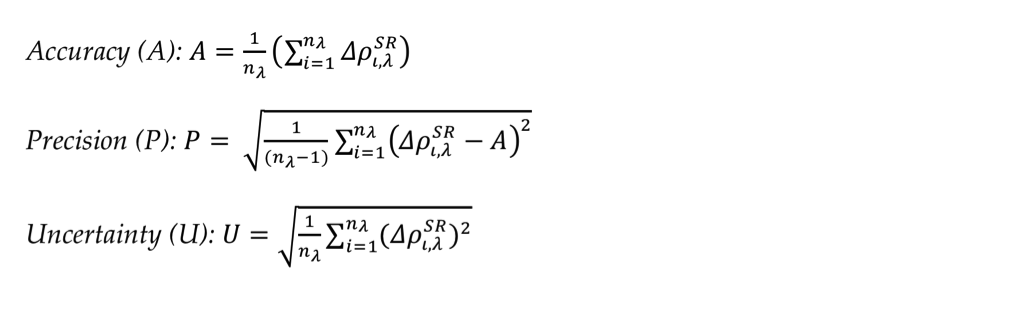## ACIX II SR

Rich Text

#### CONTACT

Surface Reflectance (SR)

─ Time series plots of the estimated SRs of every processor per site and band

─ Comparison with RadCalNET ground data per processor and band

─ Distance Matrices between the processors per sites

N x N distance matrices were created, where N is the number of AC processors. The elements of the matrix were the Euclidean distances between the retrieved averaged SRs over the 9km x 9km subsets and averaged them over the available dates.

─ Comparison with AERONET corrected data

The SRs were compared to a reference SR dataset computed by 6S radiative transfer (RT) code  and AERONET measurements. AOD, aerosol model and column water vapour were derived from  AERONET sunphotometer measurements and were used in the RT model, in order to retrieve SR  from TOA reflectance.

The residualsbetween the retrieved SRsand the reference  AERONET SRwere calculated for every pixel i, with i and ranging from 1 to nλ the total number of pixels per wavelength λ:The statistical metrics accuracy, precision and uncertainty were then estimated as below: